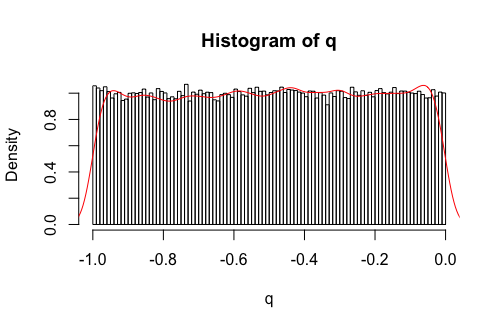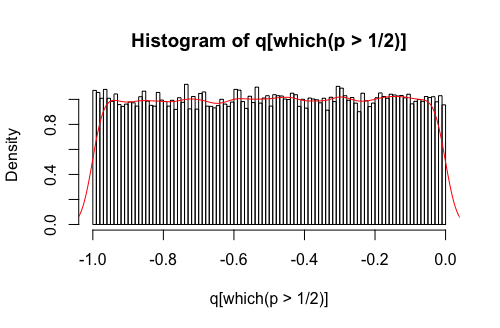### 1. cFDR method

I have made a functional_cfdr_v2 function which has the following amendments:

1. No leave-one-chromosome-out (LOCO) method

2. Use earlier density estimate of $$Q|H0$$ rather than estimating it again in the final integration step

3. Remove the contour segment in problematic low $$q$$.

• The cFDR method struggles to find contours for very low $$q$$, where there are very few data points. This results in segmented contour lines from the contourLines function (the first segment in the problematic region). Interestingly, these segmented contour lines occur only for large $$p$$.

• I remove the first segment of the segmented contours (that in the problematic low $$q$$ region), and let the contours drop straight down. James does something similar in his vly function, whereby he sets the coordinate equal to the cFDR value that we are evaluating the contour at, or the value evaluated before this point.

This function takes ~15 minutes to run for the 121,000 SNPs.

#### Testing robustness

To test the robustness of the iterations, I begin by simulating some independent $$q$$ vectors that are:

1. Sampled from the standard uniform

• The KDE (red) for $$Q$$ (estimated from $$Q,P\leq 1$$) fits the data pretty well.• As does the KDE (red) for $$Q|H0$$ (estimated by $$Q|P>1/2$$).Looking at the results over each iteration (horizontally scrollable figure), things look ok.